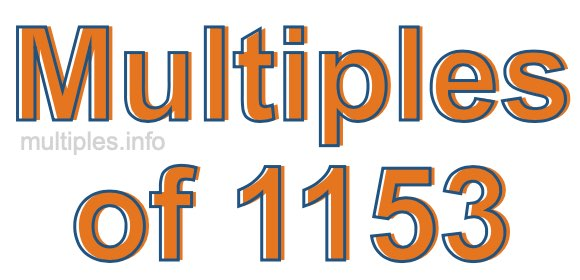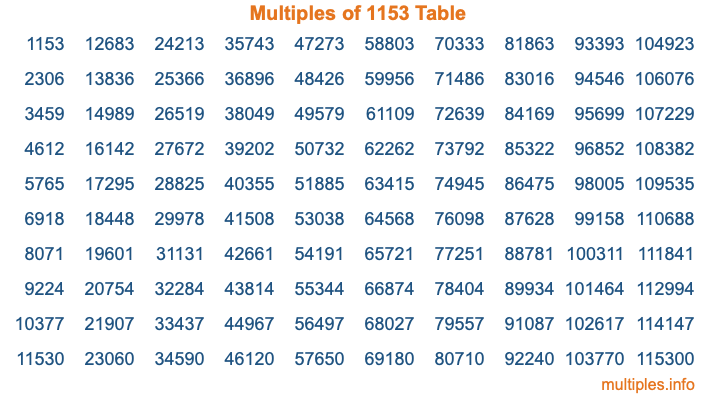Multiples of 1153Welcome to the Multiples of 1153 page. Here we will first teach you everything you will ever need to know about the multiples of 1153, and then give you a study guide summary of everything we taught you to make sure you remember it all. Use this page to look up facts and learn information about the multiples of 1153. This page will make you a multiples of one thousand one hundred fifty-three expert!

Definition of Multiples of 1153
Multiples of 1153 are all the numbers that when divided by 1153 equal an integer. Each of the multiples of 1153 are called a multiple. A multiple of 1153 is created by multiplying 1153 by an integer.

Therefore, to create a list of multiples of 1153, you start with 1 multiplied by 1153, then 2 multiplied by 1153, then 3 multiplied by 1153, and so on for as long as you want. Thus, the list of the first five multiples of 1153 is 1153, 2306, 3459, 4612, and 5765. To see a larger list of multiples of 1153, see the printable image of Multiples of 1153 further down on this page. We also have a category where you can choose any nth multiple of 1153.

Multiples of 1153 Checker
The Multiples of 1153 Checker below checks to see if any number of your choice is a multiple of 1153. In other words, it checks to see if there is any number (integer) that when multiplied by 1153 will equal your number. To do that, we divide your number by 1153. If the the quotient is an integer, then your number is a multiple of 1153.

Is  a multiple of 1153?

Least Common Multiple of 1153 and ...
A Least Common Multiple (LCM) is the lowest multiple that two or more numbers have in common. This is also called the smallest common multiple or lowest common multiple and is useful to know when you are adding our subtracting fractions. Enter one or more numbers below (1153 is already entered) to find the LCM.

Check out our LCM Calculator if you need more details about the Least Common Multiple or if you need the LCM for different numbers for adding and subtraction fractions.

nth Multiple of 1153
As we stated above, 1153 is the first multiple of 1153, 2306 is the second multiple of 1153, 3459 is the third multiple of 1153, and so on. Enter a number below to find the nth multiple of 1153.

th multiple of 1153

Multiples of 1153 vs Factors of 1153
1153 is a multiple of 1153 and a factor of 1153, but that is where the similarities end. All postive multiples of 1153 are 1153 or greater than 1153. All positive factors of 1153 are 1153 or less than 1153.

Below is the beginning list of multiples of 1153 and the factors of 1153 so you can compare:

Multiples of 1153: 1153, 2306, 3459, 4612, 5765, etc.

Factors of 1153: 1, 1153

As you can see, the multiples of 1153 are all the numbers that you can divide by 1153 to get a whole number. The factors of 1153, on the other hand, are all the whole numbers that you can multiply by another whole number to get 1153.

It's also interesting to note that if a number (x) is a factor of 1153, then 1153 will also be a multiple of that number (x).

Multiples of 1153 vs Divisors of 1153
The divisors of 1153 are all the integers that 1153 can be divided by evenly. Below is a list of the divisors of 1153.

Divisors of 1153: 1, 1153

The interesting thing to note here is that if you take any multiple of 1153 and divide it by a divisor of 1153, you will see that the quotient is an integer.

Multiples of 1153 Table
Below is an image of the first 100 multiples of 1153 in a table. The table is in chronological order, column by column. The first column has the first ten multiples of 1153, the second column has the next ten multiples of 1153, and so on.The Multiples of 1153 Table is also referred to as the 1153 Times Table or Times Table of 1153. You are welcome to print out our table for your studies.

Negative Multiples of 1153
Although not often discussed or needed in math, it is worth mentioning that you can make a list of negative multiples of 1153 by multiplying 1153 by -1, then by -2, then by -3, and so on, to get the following list of negative multiples of 1153:

-1153, -2306, -3459, -4612, -5765, etc.

Multiples of 1153 Summary
Below is a summary of important Multiples of 1153 facts that we have discussed on this page. To retain the knowledge on this page, we recommend that you read through the summary and explain to yourself or a study partner why they hold true.

There are an infinite number of multiples of 1153.

A multiple of 1153 divided by 1153 will equal a whole number.

1153 divided by a factor of 1153 equals a divisor of 1153.

The nth multiple of 1153 is n times 1153.

The largest factor of 1153 is equal to the first positive multiple of 1153.

1153 is a multiple of every factor of 1153.

1153 is a multiple of 1153.

A multiple of 1153 divided by a divisor of 1153 equals an integer.

1153 divided by a divisor of 1153 equals a factor of 1153.

Any integer times 1153 will equal a multiple of 1153.

Multiples of a Number
Here you can get the multiples of another number, all with the same attention to detail as we did for multiples of 1153 on this page.

Multiples of
Multiples of 1154
Did you find our page about multiples of one thousand one hundred fifty-three educational? Do you want more knowledge? Check out the multiples of the next number on our list!